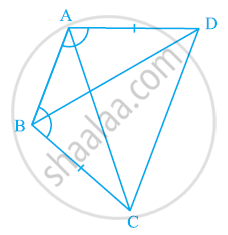Share

# ABCD is a quadrilateral in which AD = BC and ∠DAB = ∠CBA (See the given figure). Prove that - CBSE Class 9 - Mathematics

ConceptCriteria for Congruence of Triangles

#### Question

ABCD is a quadrilateral in which AD = BC and ∠DAB = ∠CBA (See the given figure). Prove that

(i) ΔABD ≅ ΔBAC

(ii) BD = AC

(iii) ∠ABD = ∠BAC.#### Solution

In ΔABD and ΔBAC,

∠DAB = ∠CBA (Given)

AB = BA (Common)

∴ ΔABD ≅ ΔBAC (By SAS congruence rule)

∴ BD = AC (By CPCT)

And, ∠ABD = ∠BAC (By CPCT)

Is there an error in this question or solution?

#### APPEARS IN

NCERT Solution for Mathematics Class 9 (2018 to Current)
Chapter 7: Triangles
Ex. 7.10 | Q: 2 | Page no. 119

#### Video TutorialsVIEW ALL 

Solution ABCD is a quadrilateral in which AD = BC and ∠DAB = ∠CBA (See the given figure). Prove that Concept: Criteria for Congruence of Triangles.
S